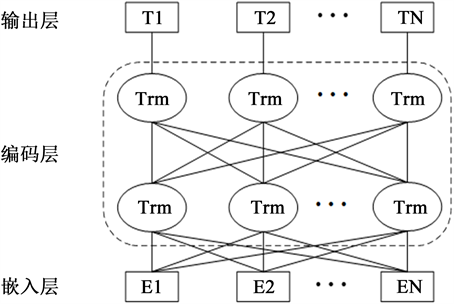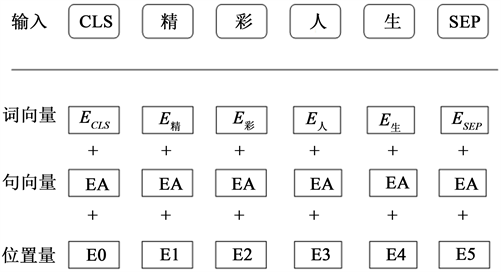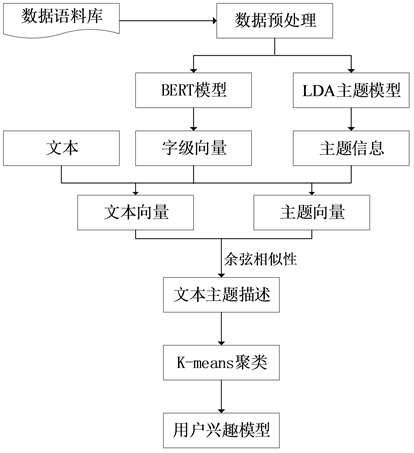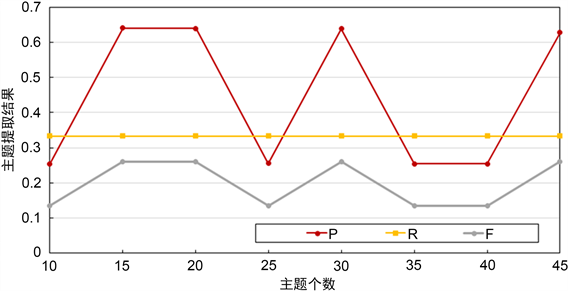#### 期刊菜单

User Interest Model Construction Method Based on BERT Model and LDA Topic Model
DOI: 10.12677/AIRR.2022.114043, PDF, HTML, XML, 下载: 57  浏览: 548  国家自然科学基金支持

Abstract: The construction of the user interest model mainly combines the user’s hobby information, travel behavior and user profile information, and comprehensively analyzes the user’s interest. As a key part of personalized information recommendation, user interest model is also an important part of personalized service. Its quality directly affects the level of personalized information recommendation services. In order to improve the quality of user interest modeling, this paper introduces word vector model and topic model to accurately express user interest, and proposes a user interest model construction method based on BERT model and LDA topic model. This method combines the BERT model and the LDA topic model. In the training process, the model can not only make full use of the context information of the entire data set, but also use LDA to obtain implicit semantic information, and at the same time extract user interests through the K-means clustering method. The experimental results show that the combined user modeling method can effectively solve the problems of sparsity and context dependence of microblog short texts. Compared with other methods, the quality of the user interest model is improved.

1. 引言

2. 相关工作

2.1. BERT模型

BERT (Bidirectional Encoder Representations from Transformers)  模型是一种与之前自然语言表示模型不同的新模型，它主要是基于双向多层的转换编码器实现的，是通过各个层中上下文信息相互联合调节的形式实现深度双向表示 。具体的结构图如图1所示。Figure 1. Structure of BERT modelFigure 2. BERT model output representation

2.2. LDA主题模型Figure 3. Schematic diagram of the LDA model

3. 方法流程及关键技术

3.1. 方法流程Figure 4. Process of user interest model construction

3.2. BERT模型的词向量表示

3.3. 主题相关度计算

${t}_{i,j}=\frac{{\phi }_{i,j}}{\underset{k=1}{\overset{h}{\sum }}{\phi }_{i,k}}$ (1)

$v\left({s}_{i}\right)={\sum }_{j=1}^{h}{t}_{i,j}v\left({t}_{{i}_{j}}\right)$ (2)

$v\left({x}_{i}\right)=\frac{{\sum }_{j=1}^{c}v\left({t}_{{i}_{j}}\right)}{c}$ (3)

$\mathrm{cos}\theta =\frac{v\left({x}_{i}\right)\cdot v\left({s}_{i}\right)}{|v\left({x}_{i}\right)|×|v\left({s}_{i}\right)|}$ (4)

$distance\left(v\left({x}_{i}\right),v\left({s}_{i}\right)\right)=0.5+0.5\ast cos\theta$ (5)

3.4. 基于K-means聚类算法的用户兴趣主题提取

4. 实验结果及分析

4.1. 实验语料与实验环境Table 1. Experimental environment settings

4.2. 实验结果分析

4.2.1. 评价指标

1) 真正类(True Positive, TP)是指原来样本为正类时，被预测为正类。

2) 假负类(False Negative, FN)是指原样本为正类时，被预测为负类。

3) 假正类(False Positive, FP)是指原样本为负类时，被预测为正类。

4) 真负类(True Negative, TN)是指原样本为负类时，被预测为负类。

1) 准确率

$P=\frac{\text{TP}}{\text{TP}+\text{FP}}$ (6)

2) 召回率

$R=\frac{\text{TP}}{\text{TP}+\text{FN}}$ (7)

3) F值

F值是对模型的综合评价指标的体现，根据准确率和召回率进行计算所得。F的计算公式如(8)所示：

$F=\frac{2\ast P\ast R}{P+R}$ (8)

4.2.2. 结果分析Figure 5. Experimental results of different subject numbersTable 2. Model comparison resultsTable 3. Mining results of user interest topicsTable 4. User-topic distribution

5. 结束语

  尚燕敏, 曹亚男, 韩毅, 李阳, 张闯. 基于主题和大众影响的用户动态行为倾向预测[J]. 计算机学报, 2018, 41(7): 1431-1447.  高永兵, 许庆瑞. 基于改进LDA模型的微博用户兴趣挖掘研究[J]. 内蒙古科技大学学报, 2019, 38(3): 272-276.  Li, H., Yan, J., Han, W., et al. (2014) Mining User Interest in Microblogs with a User-Topic Model. Communications, 11, 131-144. https://doi.org/10.1109/CC.2014.6911095  Chen, Y., Li, W., Guo, W., et al. (2015) Popular Topic Detection in Chinese Micro-Blog Based on the Modified LDA Model. 2015 12th Web Information System and Application Conference (WISA), Jinan, 11-13 September 2015, 37-42. https://doi.org/10.1109/WISA.2015.58  张霁雯, 周军. 一种基于用户兴趣特征的微博信息转发预测方法[J]. 辽宁工业大学学报(自然科学版), 2021, 41(3): 153-156+160.  杨仁凤, 陈端兵, 谢文波. 微博用户兴趣主题抽取方法[J]. 电子科技大学学报, 2018, 47(4): 633-640.  Devlin, J., et al. (2018) Bert: Pre-Training of Deep Bidirectional Transformers for Language Understanding. Proceedings of the 2019 Conference of the North American Chapter of the Association for Computational Linguistics: Human Language Technologies, Volume 1, 4171-4186.  Vaswani, A., et al. (2017) Attention Is All You Need. 31st Conference on Neural Information Processing Systems (NIPS 2017), Long Beach, 4-9 December 2017, 5998-6008.  李俊, 吕学强. 融合BERT语义加权与网络图的关键词抽取方法[J]. 计算机工程, 2020, 46(9): 89-94.  Blei, D.M., Ng, A.Y. and Jordan, M.I. (2003) Latent Dirichlet Allocation. Journal of Machine Learning Research, 3, 993-1022.  王丹. 基于主题模型的用户画像提取算法研究[D]: [硕士学位论文]. 北京: 北京工业大学, 2016.  Huang, L.F. (2013) Optimized Event Storyline Generation Based on Mixture-Event-Aspect Model. In: Proceedings of the 2013 Conference on Empirical Methods in Natural Language Processing, ACL, Washington DC, 726-735.  石元兵. 一种基于TextRank的中文自动摘要方法[J]. 通信技术, 2019, 52(9): 2233-2239.  Xue, M. (2019) A Text Retrieval Algorithm Based on the Hybrid LDA and Word2Vec Model. 2019 International Conference on Intelligent Transportation, Big Data & Smart City (ICITBS) IEEE, Changsha, 12-13 January 2019, 373-376. https://doi.org/10.1109/ICITBS.2019.00098  Wang, S., Liu, S. and Liu, Z. (2011) Research of User Interest Model Based on Ordered Pair Behavior. 2011 IEEE International Conference on Automation and Logistics (ICAL), Chongqing, 15-16 August 2011, 417-421. https://doi.org/10.1109/ICAL.2011.6024754  Liu, Q., Kai, N., He, Z., et al. (2013) Microblog User Interest Modeling Based on Feature Propagation. 2013 6th International Symposium on Computational Intelligence and Design (ISCID), Vol. 1, 383-386.